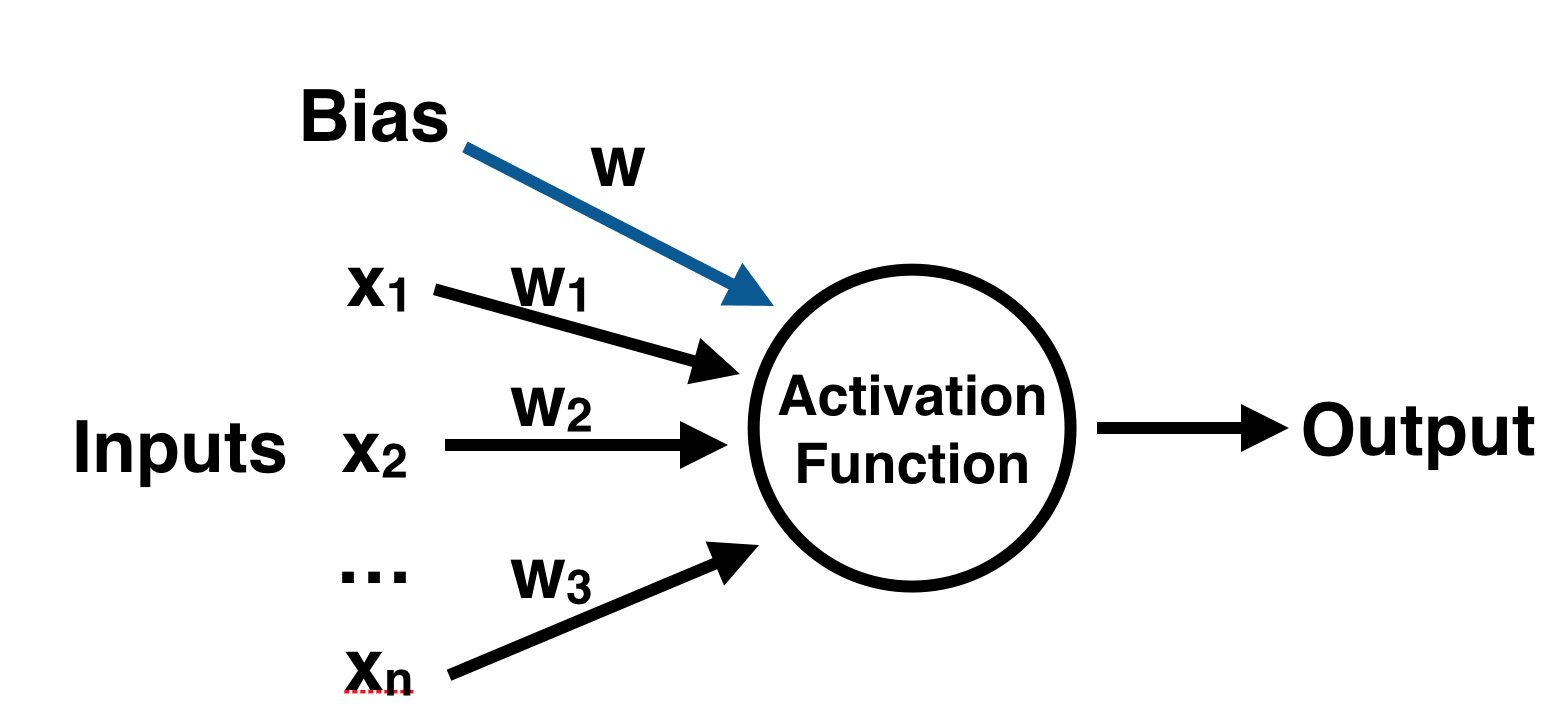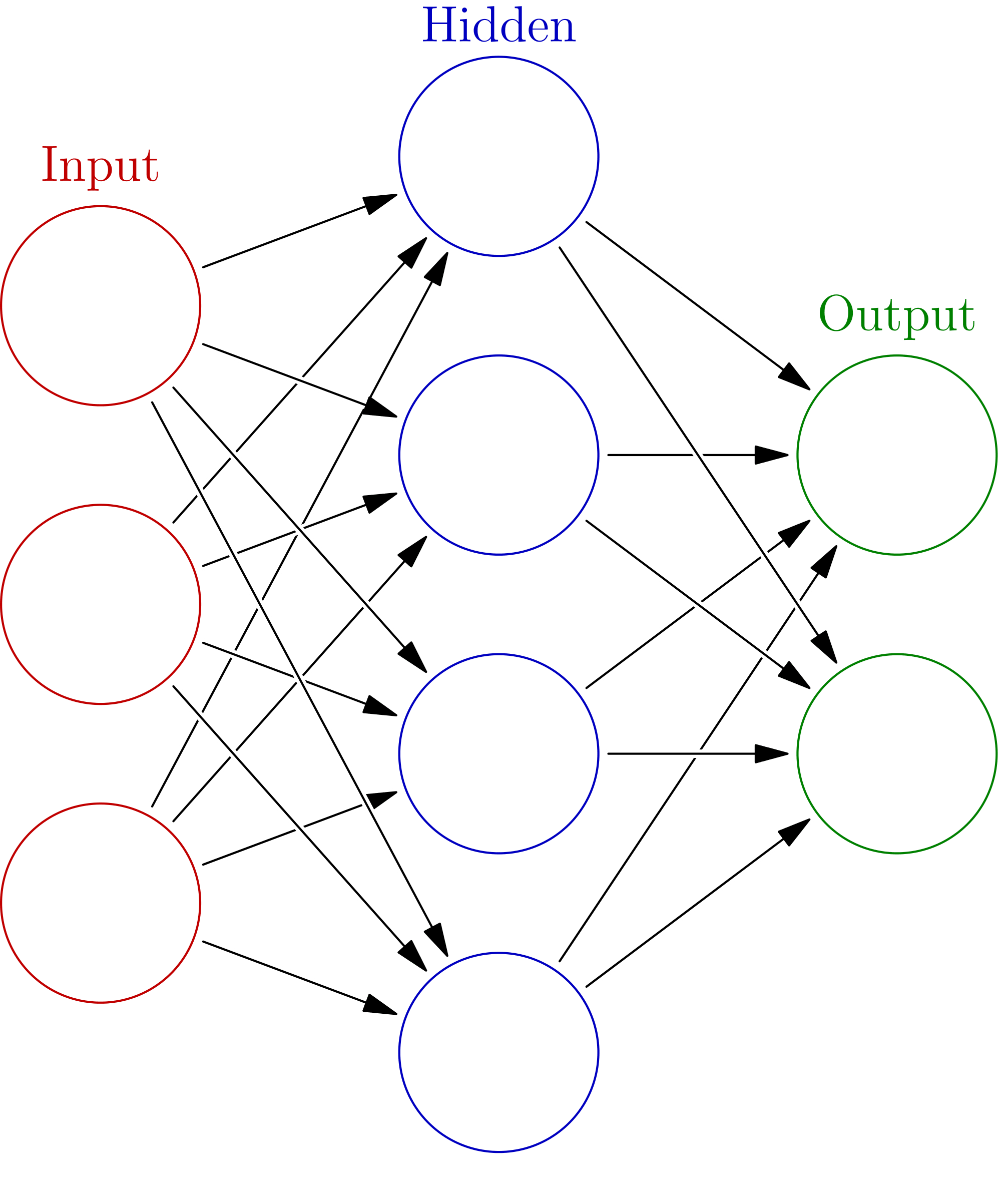• +91 7397328021                      support@deepneuron.in
My Cart 0

# Neural Network in Python

A neural network is a series of algorithms that endeavors to recognize underlying relationships in a set of data through a process that mimics the way the human brain operates. In this sense, neural networks refer to systems of neurons, either organic or artificial in nature.

## The Perceptron

Let’s start our discussion by talking about the Perceptron! A perceptron has one or more inputs, a bias, an activation function, and a single output. The perceptron receives inputs, multiplies them by some weight, and then passes them into an activation function to produce an output. There are many possible activation functions to choose from, such as the logistic function, a trigonometric function, a step function etc. We must also make sure to add a bias to the perceptron, a constant weight outside of the inputs that allows us to achieve better fit for our predictive models. Check out the diagram below for a visualization of a perceptron:Once we have the output we can compare it to a known label and adjust the weights accordingly (the weights usually start off with random initialization values). We keep repeating this process until we have reached a maximum number of allowed iterations, or an acceptable error rate.

To create a neural network, we simply begin to add layers of perceptrons together, creating a multi-layer perceptron model of a neural network. You’ll have an input layer which directly takes in your data and an output layer which will create the resulting outputs. Any layers in between are known as hidden layers because they don’t directly “see” the feature inputs within the data you feed in or the outputs. For a visualization of this check out the diagram below (source: Wikipedia).Keep in mind that due to their nature, neural networks tend to work better on GPUs than on CPU. The sci-kit learn framework isn’t built for GPU optimization. If you want to continue using GPUs and distributed models, take a look at some other frameworks, such as Google’s open sourced TensorFlow.

Let’s move on to actually creating a neural network with Python and Sci-Kit Learn!

## SciKit-Learn

In order to follow along with this tutorial, you’ll need to have the latest version of SciKit Learn (>0.18) installed! It is easily installable either through pip or conda, but you can reference the official installation documentation for complete details on this.

## Anaconda and iPython Notebook

One easy way of getting SciKit-Learn and all of the tools you need to have to do this exercise is by using Anaconda’s iPython Notebook software. This tutorial will help you get started with these tools so you can build a neural network in Python within.

## Data

For this analysis we will cover one of life’s most important topics – Wine! All joking aside, wine fraud is a very real thing. Let’s see if a Neural Network in Python can help with this problem! We will use the wine data set from the UCI Machine Learning Repository. It has various chemical features of different wines, all grown in the same region in Italy, but the data is labeled by three different possible cultivars. We will try to build a model that can classify what cultivar a wine belongs to based on its chemical features using Neural Networks. You can get the data here or find other free data sets here.

First let’s import the dataset! We’ll use the names feature of Pandas to make sure that the column names associated with the data come through.

In :
```import pandas as pd
wine = pd.read_csv('wine_data.csv', names = ["Cultivator", "Alchol", "Malic_Acid", "Ash", "Alcalinity_of_Ash", "Magnesium", "Total_phenols", "Falvanoids", "Nonflavanoid_phenols", "Proanthocyanins", "Color_intensity", "Hue", "OD280", "Proline"])```

Let’s check out the data:

In :
```wine.head()
```
Out (we’ve cut some columns from this output in the interests of formatting for this blog post — you should see more):
Cultivator Alchol Malic_Acid Ash
0 1 14.23 1.71 2.43
1 1 13.20 1.78 2.14
2 1 13.16 2.36 2.67
3 1 14.37 1.95 2.50
4 1 13.24 2.59 2.87
In :
```wine.describe().transpose()
```
Out (we’ve cut the standard deviation (std) and count columns from this output in the interests of formatting for this blog post):
mean min 25% 50% 75% max
Cultivator 1.938202 1.00 1.0000 2.000 3.0000 3.00
Alchol 13.000618 11.03 12.3625 13.050 13.6775 14.83
Malic_Acid 2.336348 0.74 1.6025 1.865 3.0825 5.80
Ash 2.366517 1.36 2.2100 2.360 2.5575 3.23
Alcalinity_of_Ash 19.494944 10.60 17.2000 19.500 21.5000 30.00
Magnesium 99.741573 70.00 88.0000 98.000 107.0000 162.00
Total_phenols 2.295112 0.98 1.7425 2.355 2.8000 3.88
Falvanoids 2.029270 0.34 1.2050 2.135 2.8750 5.08
Nonflavanoid_phenols 0.361854 0.13 0.2700 0.340 0.4375 0.66
Proanthocyanins 1.590899 0.41 1.2500 1.555 1.9500 3.58
Color_intensity 5.058090 1.28 3.2200 4.690 6.2000 13.00
Hue 0.957449 0.48 0.7825 0.965 1.1200 1.71
OD280 2.611685 1.27 1.9375 2.780 3.1700 4.00
Proline 746.893258 278.00 500.5000 673.500 985.0000 1680.00
In :
```# 178 data points with 13 features and 1 label column
wine.shape
```
Out:
`(178, 14)`

Let’s set up our Data and our Labels:

In :
```X = wine.drop('Cultivator',axis=1)
y = wine['Cultivator']```

### Train Test Split

Let’s split our data into training and testing sets, this is done easily with SciKit Learn’s train_test_split function from model_selection:

In :
```from sklearn.model_selection import train_test_split
```
In :
`X_train, X_test, y_train, y_test = train_test_split(X, y)`

## Data Preprocessing

The neural network in Python may have difficulty converging before the maximum number of iterations allowed if the data is not normalized. Multi-layer Perceptron is sensitive to feature scaling, so it is highly recommended to scale your data. Note that you must apply the same scaling to the test set for meaningful results. There are a lot of different methods for normalization of data, we will use the built-in StandardScaler for standardization.

In :
```from sklearn.preprocessing import StandardScaler
```
In :
```scaler = StandardScaler()
```
In :
```# Fit only to the training data
scaler.fit(X_train)
```
Out:
`StandardScaler(copy=True, with_mean=True, with_std=True)`
In :
```# Now apply the transformations to the data:
X_train = scaler.transform(X_train)
X_test = scaler.transform(X_test)```

## Training the model

Now it is time to train our model. SciKit Learn makes this incredibly easy, by using estimator objects. In this case we will import our estimator (the Multi-Layer Perceptron Classifier model) from the neural_network library of SciKit-Learn!

In :
`from sklearn.neural_network import MLPClassifier`

Next we create an instance of the model, there are a lot of parameters you can choose to define and customize here, we will only define the hidden_layer_sizes. For this parameter you pass in a tuple consisting of the number of neurons you want at each layer, where the nth entry in the tuple represents the number of neurons in the nth layer of the MLP model. There are many ways to choose these numbers, but for simplicity we will choose 3 layers with the same number of neurons as there are features in our data set along with 500 max iterations.

In :
`mlp = MLPClassifier(hidden_layer_sizes=(13,13,13),max_iter=500)`

Now that the model has been made we can fit the training data to our model, remember that this data has already been processed and scaled:

In :
```mlp.fit(X_train,y_train)
```
Out:
```MLPClassifier(activation='relu', alpha=0.0001, batch_size='auto', beta_1=0.9,
beta_2=0.999, early_stopping=False, epsilon=1e-08,
hidden_layer_sizes=(13, 13, 13), learning_rate='constant',
learning_rate_init=0.001, max_iter=500, momentum=0.9,
nesterovs_momentum=True, power_t=0.5, random_state=None,
verbose=False, warm_start=False)```

You can see the output that shows the default values of the other parameters in the model. I encourage you to play around with them and discover what effects they have on your neural network in Python!

## Predictions and Evaluation

Now that we have a model it is time to use it to get predictions! We can do this simply with the predict() method off of our fitted model:

In :
`predictions = mlp.predict(X_test)`

Now we can use SciKit-Learn’s built in metrics such as a classification report and confusion matrix to evaluate how well our model performed:

In :
```from sklearn.metrics import classification_report,confusion_matrix
```
In :
`print(confusion_matrix(y_test,predictions))`
```[[17  0  0]
[ 0 14  1]
[ 0  0 13]]
```
In :
`print(classification_report(y_test,predictions))`
```             precision    recall  f1-score   support

1       1.00      1.00      1.00        17
2       1.00      0.93      0.97        15
3       0.93      1.00      0.96        13

avg / total       0.98      0.98      0.98        45
```

Not bad! Looks like we only misclassified one bottle of wine in our test data! This is pretty good considering how few lines of code we had to write for our neural network in Python. The downside however to using a Multi-Layer Perceptron model is how difficult it is to interpret the model itself. The weights and biases won’t be easily interpretable in relation to which features are important to the model itself.

However, if you do want to extract the MLP weights and biases after training your model, you use its public attributes coefs_ and intercepts_.

coefs_ is a list of weight matrices, where weight matrix at index i represents the weights between layer i and layer i+1.

intercepts_ is a list of bias vectors, where the vector at index i represents the bias values added to layer i+1.

In :
```len(mlp.coefs_)
```
Out:
`4`
In :
```len(mlp.coefs_)
```
Out:
`13`
In :
```len(mlp.intercepts_)
```
Out:
`13`